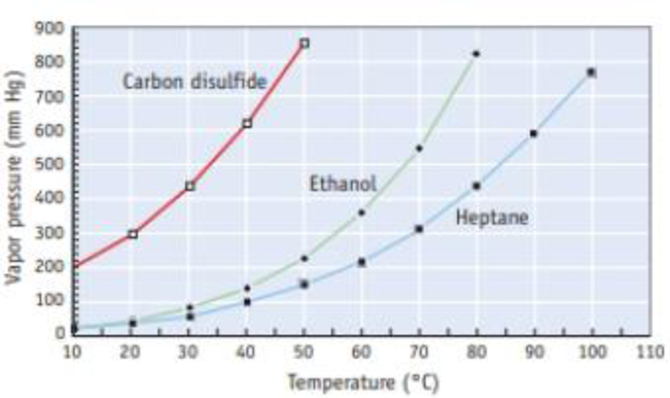# Use the vapor pressure curves illustrated here to answer the questions that follow. (a) What is the vapor pressure of ethanol, C 2 H 5 OH, at 60 °C? (b) Considering only carbon disulfide (CS 2 ) and ethanol, which has the stronger intermolecular forces in the liquid state? (c) At what temperature does heptane (C 7 H 16 ) have a vapor pressure of 500 mm Hg? (d) What are the approximate normal boiling points of each of the three substances? (e) At a pressure of 400 mm Hg and a temperature of 70 °C, is each substance a liquid, a gas, or a mixture of liquid and gas?### Chemistry & Chemical Reactivity

9th Edition
John C. Kotz + 3 others
Publisher: Cengage Learning
ISBN: 9781133949640

#### Solutions

Chapter
Section### Chemistry & Chemical Reactivity

9th Edition
John C. Kotz + 3 others
Publisher: Cengage Learning
ISBN: 9781133949640
Chapter 11, Problem 33GQ
Textbook Problem
122 views

## Use the vapor pressure curves illustrated here to answer the questions that follow.(a) What is the vapor pressure of ethanol, C2H5OH, at 60 °C? (b) Considering only carbon disulfide (CS2) and ethanol, which has the stronger intermolecular forces in the liquid state? (c) At what temperature does heptane (C7H16) have a vapor pressure of 500 mm Hg? (d) What are the approximate normal boiling points of each of the three substances? (e) At a pressure of 400 mm Hg and a temperature of 70 °C, is each substance a liquid, a gas, or a mixture of liquid and gas?

(a)

Interpretation Introduction

Interpretation: The approximate vapor pressure curve of ethanol at 60oC has to be determined.

Concept Introduction: Vapor pressure is nothing but the pressure of a vapor in contact with its liquid or solid form.

When a liquid and vapor are in equilibrium the pressure exerted by the vapor is called the equilibrium vapor pressure.

If intermolecular force is small the vapor pressure of the substance is high and the boiling point will be low.

### Explanation of Solution

The figure is,

From the figure, the vapor pressure of ethanol at

(b)

Interpretation Introduction

Interpretation: It has to be identified whether CS2 or C2H5OH has the stronger intermolecular forces in the liquid state.

Concept Introduction:

Intermolecular force: The attractive force that withholds two molecules is called as intermolecular force. The influence of intermolecular forces depends on molar mass and the functional group present in the molecule.

Dipole-Dipole Interactions: The attractive force that holds two polar molecules with help of dipole moment present in them is called as Dipole-Dipole Interactions.

The partial positive charge end of one molecule is attracted to the partial negative charge of a neighboring molecule.

Induced Dipole - Induced Dipole Forces: The attractive force that holds two nonpolar molecules with help of temporary dipole moment present in them is called as induced dipole-induced dipole forces.

(c)

Interpretation Introduction

Interpretation: The temperature at which heptane have a vapor pressure of 500mmHg has to be determined.

Concept Introduction:

Boiling point: It is the temperature at which liquid converts to vapor. At boiling point the vapor pressure of liquid and the pressure of the surroundings are equal.

Boiling point: It is the temperature at which liquid converts to vapor. At boiling point the vapor pressure of liquid and the pressure of the surroundings are equal.

(d)

Interpretation Introduction

Interpretation: The approximate normal boiling points of each of the given substances have to be determined.

Concept Introduction:

Boiling point: It is the temperature at which liquid converts to vapor. At boiling point the vapor pressure of liquid and the pressure of the surroundings are equal.

(e)

Interpretation Introduction

Interpretation: It has to be identified whether the given substances are liquid, gas or a mixture of liquid and gas at a pressure of 400mmHg and at a temperature of 70°C

Concept Introduction:

Vapor pressure is nothing but the pressure of a vapor in contact with its liquid or solid form.

When a liquid and vapor are in equilibrium the pressure exerted by the vapor is called the equilibrium vapor pressure.

### Still sussing out bartleby?

Check out a sample textbook solution.

See a sample solution

#### The Solution to Your Study Problems

Bartleby provides explanations to thousands of textbook problems written by our experts, many with advanced degrees!

Get Started

Find more solutions based on key concepts
A daily regimen of supplements and vitamin D-enriched foods increases the risk of vitamin D toxicity. T F

Nutrition: Concepts and Controversies - Standalone book (MindTap Course List)

Which elements are created in stellar nucleosynthesis?

Foundations of Astronomy (MindTap Course List)

What evolutionary advances characteristic of higher organisms are first seen in the worms?

Oceanography: An Invitation To Marine Science, Loose-leaf Versin

An object of mass m is hung from a spring and set into oscillation. The period of the oscillation is measured a...

Physics for Scientists and Engineers, Technology Update (No access codes included)